Back to Adaptive Vision Studio website

You are here: Start » Filter Reference » Feature Detection » cvHoughCircles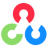# cvHoughCircles

Finds circles in a grayscale image using a Hough transform.

Name Type Range DescriptioninImage Image UINT8, single-channel input image.inDp Real 0.0 -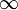Inverse ratio of the accumulator resolution to the image resolution. For example, if dp=1 , the accumulator has the same resolution as the input image. If dp=2 , the accumulator has half as big width and height.inMinDist Real 0.0 -Minimum distance between the centers of the detected circles. If the parameter is too small, multiple neighbor circles may be falsely detected in addition to a true one. If it is too large, some circles may be missed.inParam1 Real 0.0 -The higher threshold of the two passed to the Canny edge detector, the lower one is twice smaller.inParam2 Real 0.0 -The accumulator threshold for the circle centers at the detection stage. The smaller it is, the more false circles may be detected. Circles, corresponding to the larger accumulator values, will be returned first.inMinRadius Integer Minimum circle radius. When both minRadius and maxRadius equal zero, all radiuses will be accepted.inMaxRadius Integer Maximum circle radius. When both minRadius and maxRadius equal zero, all radiuses will be accepted.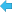outCircles Circle2DArray Output array of detected circles.

### Description

The operation detects circular objects (in pixels) in the inImage using the Hough Transform approach. It is possible to set the range of circles' radiuses using inMinRadius and inMaxRadius parameters, or accept all radiuses by setting both these parameters to 0.

### Examples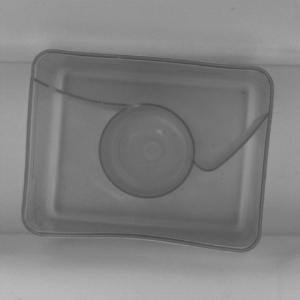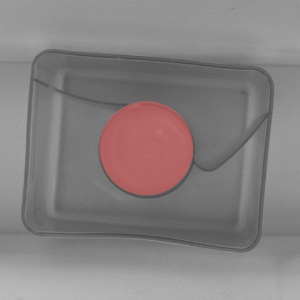cvHoughCircles performed on the sample image with inMinRadius = 40, inMaxRadius = 50.

### Remarks

The method used for circle detection is always HOUGH_GRADIENT.

Note, that first step of algorithm is calculation of gradients on image. Therefore, the input image doesn't have to be a binary image. This approach is different from the one in cvHoughLines function.

### Errors

This filter can throw an exception to report error. Read how to deal with errors in Error Handling.

List of possible exceptions:

Error type Description
DomainError Input image must be UINT8 single-channel in cvHoughCircles.

### Complexity Level

This filter is available on Basic Complexity Level.

### See Also

• cvHoughLines – Finds lines in a binary image using standard Hough transform.
• cvHoughLinesP – Finds lines segments in a binary image using probabilistic Hough transform.